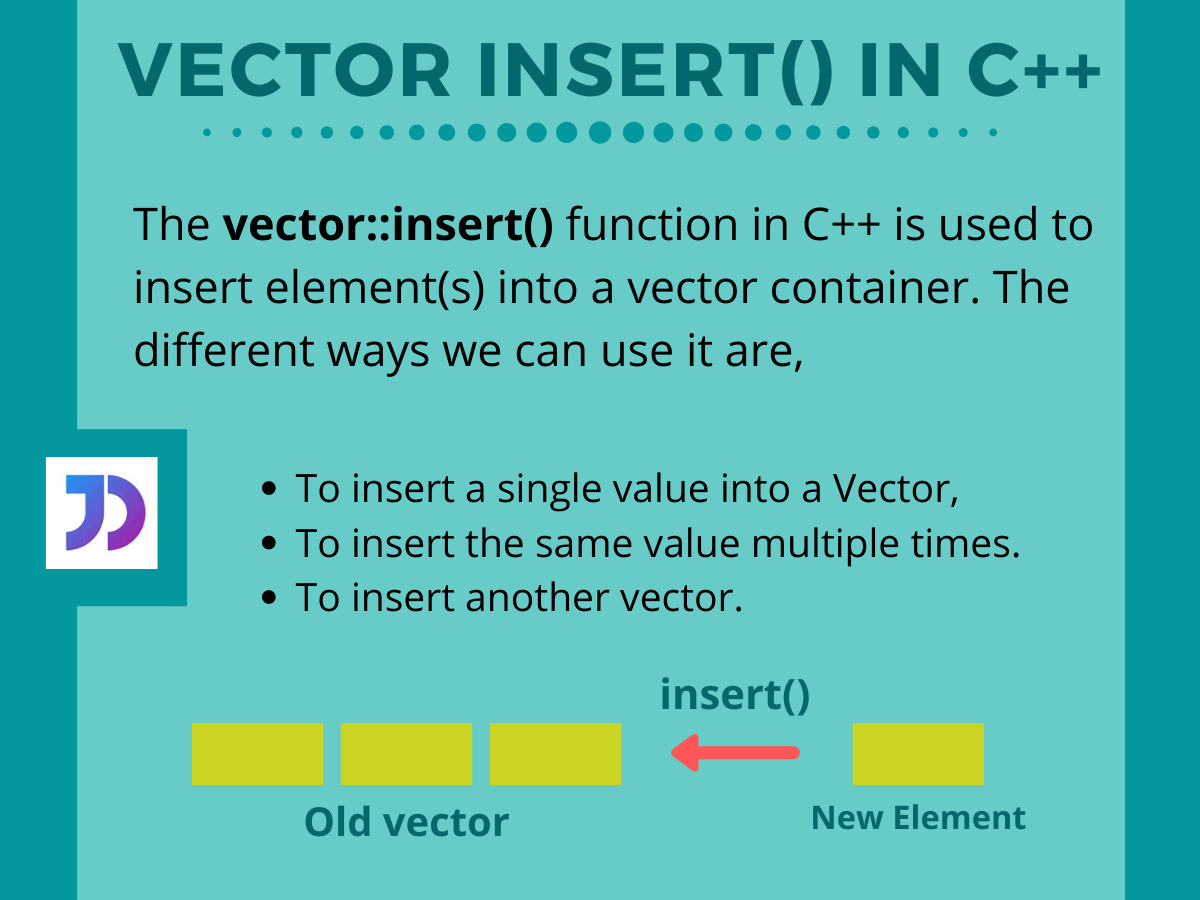# Understanding Vector insert() in C++

Published on August 3, 2022By SnehWhile we believe that this content benefits our community, we have not yet thoroughly reviewed it. If you have any suggestions for improvements, please let us know by clicking the “report an issue“ button at the bottom of the tutorial.

## Introduction

In this tutorial, we are going to learn about vector insert() in C++. As well as look at how it works and can be used to accomplish the insertion operation in different ways with examples.

## The vector::insert() function in C++

Basically, the `vector::insert()` function from the STL in C++ is used to insert elements or values into a vector container. In general, the function returns an iterator pointing to the first of the inserted elements.

## Using the insert() Function on Vectors

The `insert()` method can be used to insert single or multiple elements into a given vector in different ways, for different cases. We can insert a single value at our desired position, we can even insert multiple values into the vector at once, and even we can insert a bunch of values from another vector to it.

So, let us see how we can do that with ease.

### 1. Insert a single value into a Vector

We can directly pass an iterator pointing to our desired position and the value to be inserted there to the `insert()` function to modify a vector.

Look carefully at the example below, here we try to insert a value 10 at the beginning of the vector.

``````#include<iostream>
#include<vector>
using namespace std;
int main()
{
vector<int> vec {1,2,3,4,5};

cout<<"Intially vector: ";
for(auto i=vec.begin(); i<vec.end(); i++)
{
cout<<" "<<*i;
}

vec.insert(vec.begin(),10);//Inserting 10 to the vector

cout<<"\n\nThe modified vector is: ";
for(auto i=vec.begin(); i<vec.end(); i++)
{
cout<<" "<<*i;
}
return 0;
}
``````

Output;

``````Intially vector:  1 2 3 4 5

The modified vector is:  10 1 2 3 4 5
``````

Here,

• Firstly we initialize a vector, `vec`. And print the same,
• Then we call the insert() function on the vector `vec` with parameters `vec.begin()` and 10(new value). Note, here `vec.begin()` returns an iterator pointing to the start of the vector,
• After the insertion has been done we print the new vector using a simple for loop to see the resultant vector.

### 2. Insert the same value Multiple times

We can also insert multiple values to a vector at once using the `insert()` function. This can be done by passing an iterator pointing to our starting position where we want to insert, the number of times the value is going to repeat, and at last the value.

The example below illustrates the use properly.

``````#include<iostream>
#include<vector>
using namespace std;
int main()
{
vector<int> vec {10,20,30,40};

cout<<"Intially vector: ";
for(auto i=vec.begin(); i<vec.end(); i++)
{
cout<<" "<<*i;
}

vec.insert(vec.end(),3,100);//Inserting 100, 3 times to the vector

cout<<"\n\nThe modified vector is: ";
for(auto i=vec.begin(); i<vec.end(); i++)
{
cout<<" "<<*i;
}
return 0;
}
``````

Output;

``````Intially vector:  10 20 30 40

The modified vector is:  10 20 30 40 100 100 100
``````

Here,

• We initialize our vector `vec` and print the same,
• Then we pass an iterator pointing to the end of the vector, as returned by `vec.end()`, 3(the number of times we want the value to repeat), and the value 100 to the insert() function.
• In this way, as we can observe from the output, 100 is inserted thrice at the end of the vector, `vec`.

### 3. Insert Another Vector

Further, we can also insert elements of another vector to our old vector. Just we need to pass an iterator pointing to the position in our vector where we need to insert another vector. Along with that, the iterators pointing to the starting and end of the second vector.

Let us take a small example to understand the working.

``````#include<iostream>
#include<vector>
using namespace std;
int main()
{
vector<int> vec {2,4,6,8};
vector<int> vec2 {1,3,5,7};
cout<<"Intially first vector: ";
for(auto i=vec.begin(); i<vec.end(); i++)
{
cout<<" "<<*i;
}
cout<<"\nIntially second vector: ";
for(auto i=vec2.begin(); i<vec2.end(); i++)
{
cout<<" "<<*i;
}

//Inserting vec2 at the beginning of the vec vector
vec.insert(vec.begin(),vec2.begin(),vec2.end());

cout<<"\n\nThe modified vector is: ";
for(auto i=vec.begin(); i<vec.end(); i++)
{
cout<<" "<<*i;
}
return 0;
}
``````

Output;

``````Intially first vector:  2 4 6 8
Intially second vector:  1 3 5 7

The modified vector is:  1 3 5 7 2 4 6 8
``````

Here, `vec` and `vec2` are two vectors. Out of which vec2 is the one whose elements we need to insert into the vector, vec. We call the `insert()` function with appropriate parameters as mentioned earlier. This modifies our vector `vec`, resulting in the insertion of the second vector elements at the beginning.

## Conclusion

So, in this tutorial, we explained the working as well as the use of the vector insert() function from the STL in C++. For better understanding, we recommend trying the above code snippets yourselves. And for any questions, feel free to comment below.

Click below to sign up and get \$200 of credit to try our products over 60 days!

### Popular Topics##### Hollie's Hub for Good

Working on improving health and education, reducing inequality, and spurring economic growth? We’d like to help.##### Become a contributor

You get paid; we donate to tech nonprofits.

##### Welcome to the developer cloud

DigitalOcean makes it simple to launch in the cloud and scale up as you grow – whether you’re running one virtual machine or ten thousand.© 2023 DigitalOcean, LLC.